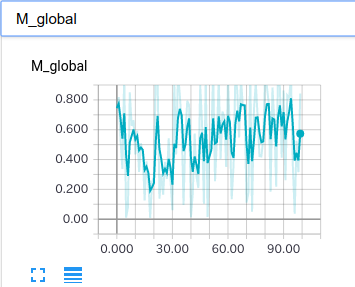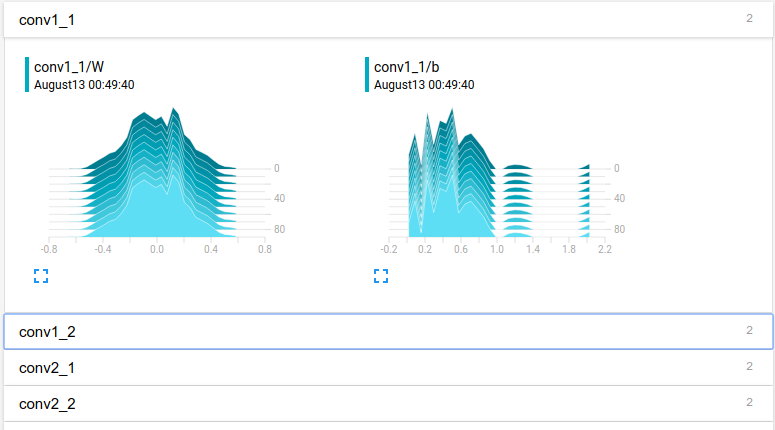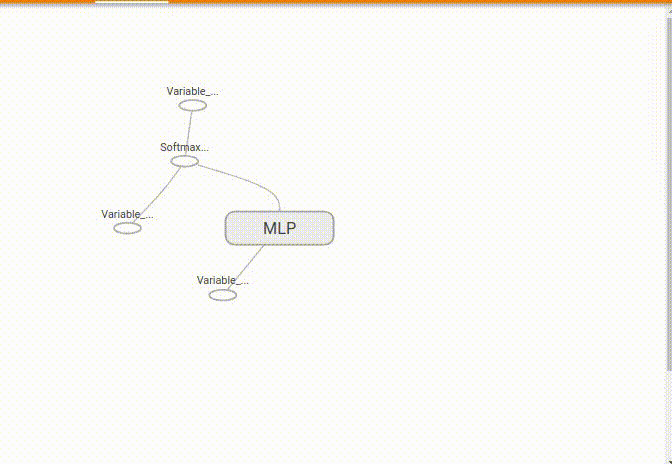Log TensorBoard events with chainer

## Project description# tensorboard-chainer

Write tensorboard events with simple command. including scalar, image, histogram, audio, text, graph and embedding.

This is based on tensorboard-pytorch.

## Usage

Install tensorflow.

pip install tensorflow


Execute demo.py and tensorboard. Access "localhost:6006" in your browser.

cd examples
python demo.py
tensorboard --logdir runs


## Scalar example## Histogram example## Graph example## Name scope

Like tensorflow, nodes in the graph can be grouped together in the namespace to make it easy to see.

import chainer
import chainer.functions as F
from tb_chainer import name_scope, within_name_scope

class MLP(chainer.Chain):
def __init__(self, n_units, n_out):
super(MLP, self).__init__()
with self.init_scope():
self.l1 = L.Linear(None, n_units)  # n_in -> n_units
self.l2 = L.Linear(None, n_units)  # n_units -> n_units
self.l3 = L.Linear(None, n_out)  # n_units -> n_out

@within_name_scope('MLP')
def __call__(self, x):
with name_scope('linear1', self.l1.params()):
h1 = F.relu(self.l1(x))
with name_scope('linear2', self.l2.params()):
h2 = F.relu(self.l2(h1))
with name_scope('linear3', self.l3.params()):
o = self.l3(h2)
return o


How to save the logs using this model is shown below. add_all_variable_images is the function that saves the Variable's data in the model that matches the pattern as an images. add_all_parameter_histograms is the function that save histograms of the Parameter's data in the model that match the pattern.

from datetime import datetime
from tb_chainer import SummaryWriter

model = L.Classifier(MLP(1000, 10))

res = model(chainer.Variable(np.random.rand(1, 784).astype(np.float32)),
chainer.Variable(np.random.rand(1).astype(np.int32)))

writer = SummaryWriter('runs/'+datetime.now().strftime('%B%d  %H:%M:%S'))

writer.close()


## Project details

This version0.5.30.5.20.4.0

Uploaded source